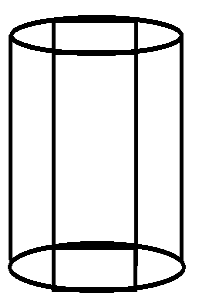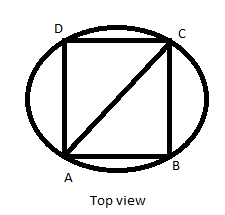QUESTION

# The trunk of a tree is a right circular cylinder 1.5m in radius and 10m high. What is the volume of timber which remains when the trunk is trimmed just enough to reduce it to a rectangular parallelogram on a square base.A. 45${m^3}$B. 54${m^3}$C. 50${m^3}$D. 48${m^3}$

Hint – In such questions we need to visualize the solid figure and make a rough figure in order to observe the required quantity and way to obtain it as in this question we need to know the basic formulae of volume of solid figures.Given,
In $\Delta ABC$
Radius of trunk=$\sqrt {2{{(side)}^2}}$
$A{C^2} = A{B^2} + B{C^2}^{} \\ {(2R)^2} = 2{(AB)^2} \\ 4{R^2} = 2A{B^2} \\ 4 \times {(1.5)^2} = 2A{B^2} \\ A{B^2} = 2 \times 2.25 \\ AB = 2.12m \\$
Area of square= ${(side)^2}$ =${(2.12)^2} \\ = 4.49{m^2} \\$
=44.9${m^3}$
$\cong 45{m^3}$
Hence the answer to this question is $45{m^3}$.